Foxtable(狐表)用户栏目专家坐堂 → [求助]怎样从类似的excel表格把数据批量导入狐表

共有1012人关注过本帖树形打印复制链接

# 主题：[求助]怎样从类似的excel表格把数据批量导入狐表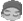qjm107
1楼 | 信息 | 搜索 | 邮箱 | 主页 | UC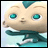加好友发短信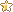[求助]怎样从类似的excel表格把数据批量导入狐表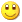Post By：2020/1/10 21:41:00 [只看该作者]

 下载信息  [文件大小：   下载次数： ]点击浏览该文件:报名表张三.xlsx

 下载信息  [文件大小：   下载次数： ]点击浏览该文件:报名表王五.xlsx

大家好，我现在有一批excel表格，它们都有一个共同特点，若某个单元格的内容是“姓名：”，它右边一个单元格的内容就是报名人员的姓名，如张三、李四等，现在我想把这些姓名数据都导入狐表，如附件中所示，请问代码怎样编写？之前讨论过的类似代码如下，要求所有的姓名都在excel的同一个位置的单元格，才能实现，而此次我上传的这3个excel中，姓名都不在excel的同一位置的单元格。
For Each file As String In filesys .GetFiles("f:\报名表")
If
file.EndsWith(".xls") OrElse file.EndsWith(".xlsx") Then
Dim
Book As New XLS.Book(file)
Dim
Sheet As XLS.Sheet = Book.Sheets(0)
Dim

dr("姓名") = sheet(1
 下载信息  [文件大小：   下载次数： ]点击浏览该文件:报名表李四.xlsx

,1).Text
End If
Next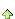qjm107
2楼 | 信息 | 搜索 | 邮箱 | 主页 | UC加好友发短信Post By：2020/1/10 21:41:00 [只看该作者]

 下载信息  [文件大小：   下载次数： ]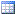点击浏览该文件:报名表.tableqjm107
3楼 | 信息 | 搜索 | 邮箱 | 主页 | UC加好友发短信Post By：2020/1/10 21:43:00 [只看该作者]

 之前讨论的如此链接所示，参考：http://www.foxtable.com/webhelp/scr/2492.htm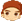4楼 | 信息 | 搜索 | 邮箱 | 主页 | UC加好友发短信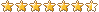Post By：2020/1/10 22:18:00 [只看该作者]

 如果每个表格的格式都不一样，只能是给每个表格写一段对应的导入代码。qjm107
5楼 | 信息 | 搜索 | 邮箱 | 主页 | UC加好友发短信Post By：2020/1/12 21:36:00 [只看该作者]

 其实，我想咨询的是，如果这一批excel表格，它们都有一个共同特点，若某个单元格的内容是“姓名：”，它右边一个单元格的内容就是报名人员的具体姓名。能否依据这个共同特点，运用人工智能或类似人工智能技术的编程代码来实现导入？6楼 | 信息 | 搜索 | 邮箱 | 主页 | UC加好友发短信Post By：2020/1/12 22:29:00 [只看该作者]

 遍历所有单元格，如果某个 单元格值为“姓名：”，则取右边一个单元格值填入姓名列，类似Dim Book As New XLS.Book("c:\test\订单.xls")Dim Sheet As XLS.Sheet = Book.Sheets(0)Tables("订单").StopRedraw()Dim r As Row = Tables("订单").AddNew()For n As Integer = 0 To Sheet.Rows.Count -1     For m As Integer = 0 To Sheet.Cols.Count- 1      if Sheet(n,m).Value = “姓名：” then r("姓名") = Sheet(n,m+1).Valueend if    NextNextTables("订单").ResumeRedraw()qjm107
7楼 | 信息 | 搜索 | 邮箱 | 主页 | UC加好友发短信Post By：2020/1/14 21:15:00 [只看该作者]

 各位专家们关于上面的类似代码，我也写了如下一段去试验，但是，有错误提示信息，并且也出不来结果，请问需要怎么修改。注：3个类似的excel表格，我已放入计算机F盘的“报名”文件夹里了。请问代码还需怎么修改？For Each file As String In filesys .GetFiles("f:\报名")If file.EndsWith(".xls") OrElse file.EndsWith(".xlsx") ThenDim Book As New XLS.Book(file)Dim Sheet As XLS.Sheet = Book.Sheets(0)Tables("报名表").StopRedraw()Dim r As Row = Tables("报名表").AddNew()For n As Integer = 0 To Sheet.Rows.Count -1    For m As Integer = 0 To Sheet.Cols.Count- 1        If Sheet(n,m).Value = "姓名:" Then            r("姓名") = Sheet(n,m+1).Value        End If    NextNextTables("报名表").ResumeRedraw()8楼 | 信息 | 搜索 | 邮箱 | 主页 | UC加好友发短信Post By：2020/1/14 21:58:00 [只看该作者]

 Tables("报名表").StopRedraw()For Each file As String In filesys .GetFiles("f:\报名")    If file.EndsWith(".xls") OrElse file.EndsWith(".xlsx") Then        Dim Book As New XLS.Book(file)        Dim Sheet As XLS.Sheet = Book.Sheets(0)        ‘’Output.Show(Sheet.Rows.Count & "," & Sheet.Cols.Count)        Dim r As Row = Tables("报名表").AddNew()        For n As Integer = 0 To Sheet.Rows.Count -1            For m As Integer = 0 To Sheet.Cols.Count- 1        ‘’Output.Show(Sheet(n,m).Value )                If Sheet(n,m).Value = "姓名:" Then                    r("姓名") = Sheet(n,m+1).Value                End If            Next        Next    End IfNextTables("报名表").ResumeRedraw()qjm107
9楼 | 信息 | 搜索 | 邮箱 | 主页 | UC加好友发短信Post By：2020/1/16 21:57:00 [只看该作者]

 谢谢你，有点蓝，姓名这一列的结果能出来了，但在狐表中，姓名这一列的右边还有“性别”和“身份证号”列，同样，在对应的Excel中，张三、李四、王五的真实性别和身份证号码也都在左边单元格的值分别为“性别：”和“身份证号：”的相应单元格中，请问，在此种情况下，要同时提取，对应的Excel中，张三、李四、王五等这一批人的性别和身份证号，代码需怎样修改，我修改的代码如下，但结果出不来，请问，代码存在哪儿，还需修改哪儿？Tables("报名表").StopRedraw()For Each file As String In filesys .GetFiles("f:\报名")    If file.EndsWith(".xls") OrElse file.EndsWith(".xlsx") Then        Dim Book As New XLS.Book(file)        Dim Sheet As XLS.Sheet = Book.Sheets(0)        ''Output.Show(Sheet.Rows.Count & "," & Sheet.Cols.Count)        Dim r As Row = Tables("报名表").AddNew()        For n As Integer = 0 To Sheet.Rows.Count -1            For m As Integer = 0 To Sheet.Cols.Count- 1                ''Output.Show(Sheet(n,m).Value )                If Sheet(n,m).Value = "姓名:" Then                    r("姓名") = Sheet(n,m+1).Value                    If Sheet(n,m).Value = "性别:" Then                        r("性别") = Sheet(n,m+1).Value                        If Sheet(n,m).Value = "身份证号:" Then                            r("身份证号") = Sheet(n,m+1).Value                        End If                      Next                End If            Next            Next    End IfNextEnd IfNextTables("报名表").ResumeRedraw()qjm107
10楼 | 信息 | 搜索 | 邮箱 | 主页 | UC加好友发短信Post By：2020/1/16 21:58:00 [只看该作者]

 下载信息  [文件大小：   下载次数： ]点击浏览该文件:报名表王五.xlsx

 下载信息  [文件大小：   下载次数： ]点击浏览该文件:报名表李四.xlsx

 下载信息  [文件大小：   下载次数： ]点击浏览该文件:报名表张三.xlsx总数 20 1 2 下一页